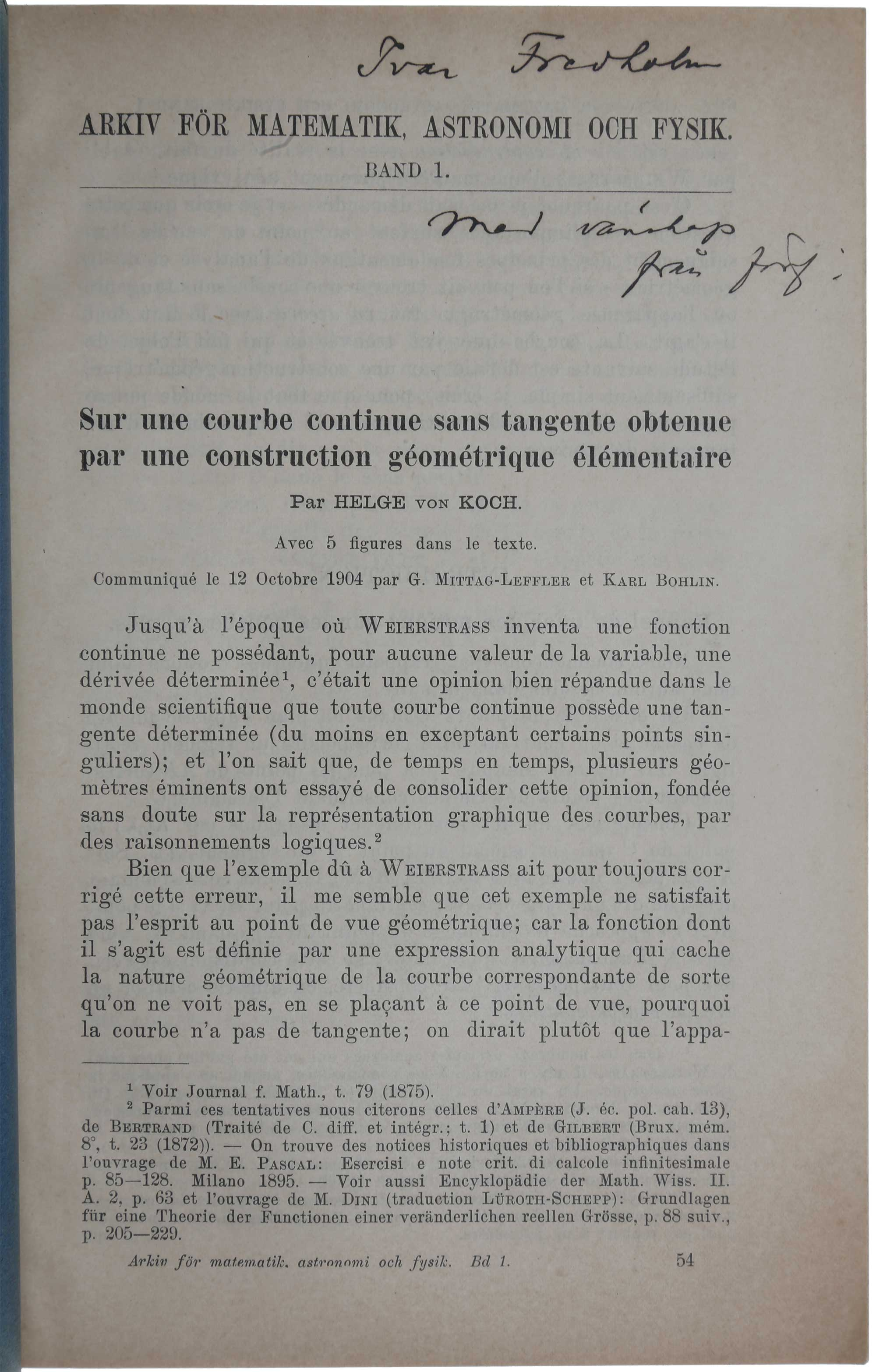## Sur une courbe continue sans tangente, obtenue par une construction géométrique élémentaire.

Stockholm: P.A. Norstedt & Soner, 1904.

First edition, extremely rare offprint issue, inscribed by the author to Fredholm, of von Koch’s paper, which contains the first examples of geometric fractals, the famous ‘Koch curve’ and ‘Koch snowflake’. The term ‘fractal’ was coined much later, by Benoit Mandelbrot in his 1975 book Les objets fractals, forme, hasard et dimension. A fractal is an object that displays ‘self-similarity’ on all scales; it need not exhibit exactly the same structure at all scales, but the same ‘type’ of structures must appear on all scales. A fractal should also have a ‘dimension’ that is not a whole number, unlike a line (dimension 1) or a plane (dimension 2). Today fractals have found a bewildering variety of applications in both the arts and sciences (fractal patterns have been found in the works of Jackson Pollock).

The first example of a fractal was the ‘Cantor set,’ introduced by Georg Cantor (1845-1918) in 1883. This set is obtained by starting with a line segment and then removing the middle third, leaving two shorter line segments; then one removes the middle third of each of these two segments; iterating the process indefinitely results in the Cantor set, an infinite set of points that is ‘self-similar,’ in the sense that if one magnifies a section of the set, one obtains the whole set again. The Cantor set is not really a curve: it is an infinite set of points, but is ‘countable’, like the whole numbers 1,2,3,…, but unlike the original line segment whose points cannot be ‘counted’ in this way (a fact that was also proved by Cantor). In this sense the Cantor set cannot be regarded as a geometric fractal.

Koch modified Cantor’s construction to obtain a genuine curve. The Koch curve is constructed by starting with a straight line segment, erecting an equilateral triangle with base the middle third of the original segment, and then erasing that middle third. This produces a shape with four line segments, each one-third the length of the original segment. The same construction is now applied to each of these four segments. The Koch curve is the result of iterating this process indefinitely. The Koch snowflake is the result of applying the process to an equilateral triangle instead of a line segment, so that a Koch snowflake is three Koch curves joined together. The Koch curve is self-similar, in the sense that any part of it, when magnified, looks the same as part of the original curve. The Koch curve has infinite length, because the total length increases by a factor 4/3 with each iteration, so that after n iterations the length is increased by a factor (4/3)n, which becomes larger without limit as n increases. The ‘fractal dimension’ of the Koch curve maybe be calculated by counting the number N(d) of circles of diameter d needed to cover the curve; as d get smaller N(d) gets larger, but the ratio – log N(d) / log d approaches a definite number as d approaches zero. In fact, it approaches log 4/log 3 = 1.26186 - the fractal dimension of the Koch curve.

Koch’s aim in this paper was not, of course, to give examples of fractals, whose definition was 70 years in the future. Rather, as the title of the paper states, he wished to give a geometric construction of a curve that was continuous but not differentiable, that is, it had no well-defined tangent at any point. The first example of such a curve had been given by Karl Weierstrass (1815-97) in a paper presented on 18 July 1872 to the Prussian Academy of Sciences (it was not published until 1895). Before Weierstrass most mathematicians believed that continuous curves were differentiable except at isolated points. But Koch was dissatisfied with Weierstrass’s example: in the introduction to the present paper, he wrote, “it seems to me that his example is not satisfactory from the geometrical point of view since the function is defined by an analytic expression that hides the geometrical nature of the corresponding curve and so from this point of view one does not see why the curve has no tangent. Rather it seems that the appearance is actually in contradiction with the factual reality established by Weierstrass in a purely analytic way.” Koch showed that his own curve, which was constructed in terms of elementary geometry, also had the property of being continuous everywhere but differentiable nowhere. Moreover, while the Koch curve is a fractal, Weiertsrass’s curve is not, as it is not self-similar (although it does have a dimension between 1 and 2).

Von Koch (1870-1924) “was a student of Gösta Mittag-Leffler and succeeded him as professor of mathematics at Stockholm University in 1911. His first work was on the theory of determinants of infinite matrices, a topic initiated by the French mathematician Henri Poincaré. This work now forms part of the theory of linear operators, which are fundamental in the study of quantum mechanics” (Britannica). Fredholm was also a student of Mittag-Leffler, and worked at Stockholm University for the rest of his life, becoming professor of mathematical physics there in 1906. Fredholm “solved, under quite broad hypotheses, a very general class of integral equations that had been the subject of extensive research for almost a century. His work led indirectly to the development of Hilbert spaces and so to other more general function spaces” (DSB, under Fredholm). Referring to his work on integral equations, “in an address to the Swedish Congress of Mathematics (1909) [Fredholm] said, ‘the works of my colleague von Koch on infinite determinants greatly facilitated my research ‘” (Stewart, Fredholm, Hilbert, Schmidt: three fundamental papers on integral equations (2011)).

Offprint from Arkiv för matematik 1 (1904). 8vo (215 x 139 mm), pp. 681-704. Original printed wrappers, a little chipping with small loss to left upper corner and lower right.

Item #3660

Price: \$3,850.00